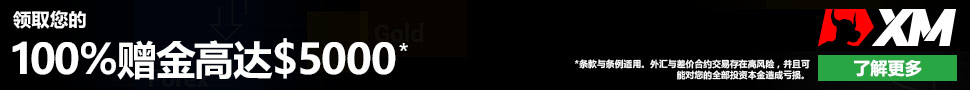# 12.2 不同类型的交易风格与交易系统```Input : Length ( 10 )
If Close > High( High , Length ）〔l〕 Then Buy on Close```

“输人变数：长度（10 ) ”这让你很容易调整最高价的回顾期间长度。一套系统的所有输人变数都摆在最顶端。〔 1 〕 是指期间长度是由前一期起算，不是由当期起算。由于在收盘之前，你不能确定当期的最高价究竟是多少，所以不能把当期最高价考虑在信号设定内。

`If High > High ( High , Length ）〔 1 〕 Then Buy`

`If C >High( H,10）〔l〕＋5 Points Then Buy on C`

```Buffer = Stedev ( Close , 10 ）〔 1 〕
If C>High(H,10）〔l〕＋Buffer Then Buy on C```

`If Close > Average ( Close , 35 ) And Close 〔 1 〕 ＞Average ( Close , 35 ) [ l 〕 Then Buy`

```Inputs ：Length(10) , Lengthv ( 5 )
Condition1=High > Highest ( High , Length ）〔l〕
Condition2=Volume>(Average ( Volume , Lengthv ) * 1.25 )
If Conditionl And Condition2 Then Buy On Closer```

```Input : Length1( 10 ) , Length2( 35 )
If Average ( Close , Length1) Cross Over
Average ( Close , Length2) Then Buy On Close```

10 期均线向上穿越35 期均线，代表买进信号。虽然信号发生在当期结束，但可以在下一期开盘时采取行动，因为在当期还没有收盘之前，无法十分确定包含当期收盘价在内的移动平均数值。有时移动平均看起来似乎会穿越，但临收盘前可能突然发生逆转。你也可以对其稍微改变，利用前一期资料而在当期开盘买进：

```Input : Length1( 10 ) , Length2( 35 )
If Average ( Close , Length1) 〔l〕 Cross Over
Average ( Close , Length2) Then Buy On Open```

```Input:BarsBack(10)
Condition1=Average(C，50)>Average(C，50)〔BarsBack〕```

```Input:Length2(35)，ATRlen(10)
Condition2=[C-Average(C，Length2)]<ATR[ATRlen(10)]```

`Tnput:Length(14)，BuyZone(30) If Slowk(Length)>S1owD(Length)and S1owD(Length)Crosses Above BuyZone Then Buy On Close`

```Input:RSILen(10)，BuyZone(30)

```Input : SellZone ( 70 )
Condition1 = SlowD ( Length ) < SellZone```

`If ADX ( 10 ) > 30 , And SlowD ( 14 ) > 85 , Then Buy On Close`• QQ咨询
• 回顶